# Quadrilaterals - Perimeters and Areas

Go back to  'Mensuration'

We start with the simplest case. Consider a rectangle of dimensions $$l \times b:$$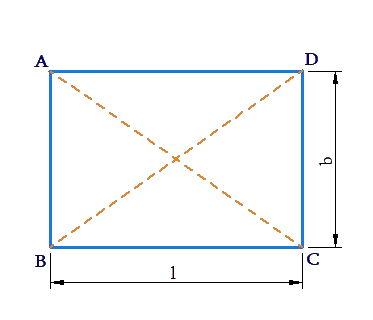The perimeter of ABCD is

$AB + BC + CD + DA = 2(l + b)\; \rm{units}$

The area is

$A = (l \times b)\; \rm{sq.\;units}$

AC and BD  are the diagonal;  their lengths can be evaluated using the Pythagoras theorem:

$AC = BD = \sqrt {{l^2} + {b^2}}$

A square is a special case of a rectangle in which length is equal to breadth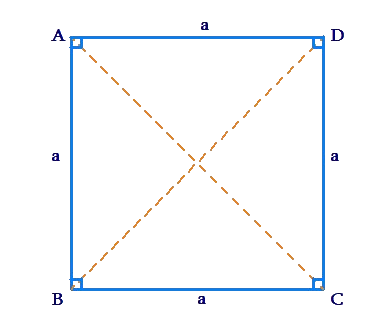For this square ABCD,

• $$\text{Perimeter}\,= 4a\,{\rm{ units}}$$
• $${\rm{Area = }}{{\rm{a}}^2}\;{\rm{ sq.}}\;{\rm{units}}$$
• $$AC = BD = \sqrt 2 a\;{\rm{ units}}$$

Next, consider a parallelogram: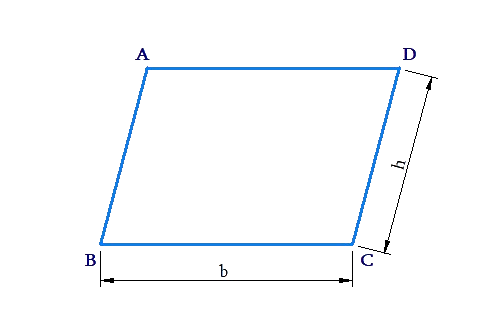We  have shown earlier that the area of a parallelogram equals base X height . For the figure above,

$\text{Area}\left( {ABCD} \right) = b \times h$

The perimeter P can be calculated as follows:

\begin{align}&P\; = AB + BC + CD + DA\\ &\quad = 2(AB + BC)\end{align}

Suppose that the parallelogram is a rhombus. Recall  that the diagonals of a rhombus are perpendicular, as shown in the following figure: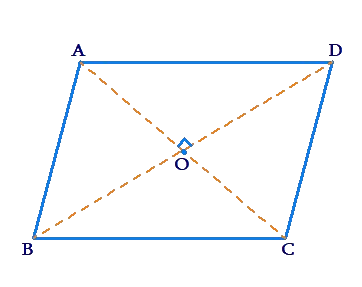Suppose that the diagonal lengths are AC=dunits  and BD =dunits. We note that the diagonals divide the rhombus into four triangles of equal area, and so

\begin{align}&\rm{area}\,(ABCD) = 4\,area(\Delta AOD)\\&\qquad\qquad\qquad = 4\left( {\frac{1}{2} \times OD \times OA} \right)\\&\qquad\qquad\qquad = 4\left( {\frac{1}{2} \times \frac{{BD}}{2} \times \frac{{AC}}{2}} \right)\\&\qquad\qquad\qquad = \frac{1}{2} \times {d_1} \times {d_2}\,{\rm{ sq }}\,{\rm{.units}}\end{align}

Thus, the area of a rhombus  equals  half the product of its diagonals.

Another particular kind of quadrilateral is a trapezium, a quadrilateral in which two sides are parallel :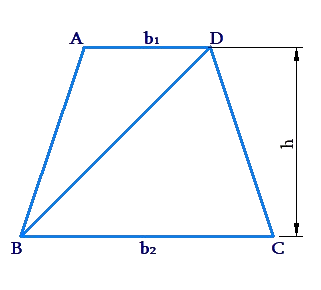We know the base lengths (the lengths of the parallel sides) and the height of the trapezium (the distance between the parallel sides). Can we express the area of the trapezium in terms of these parameters?

Consider $$\Delta ABD$$ and $$\Delta DBC$$  separately.

We have

\begin{align}&\rm{area}\left( {\Delta ABD} \right) = \frac{1}{2} \times AD \times height\\ & \qquad\qquad\qquad\;= \frac{1}{2} \times {b_1} \times h\\&\rm{area}\left( {\Delta DBC} \right) = \frac{1}{2} \times BC \times height\\ &\qquad\qquad\qquad\;= \frac{1}{2} \times {b_2} \times h\end{align}

Thus,

\begin{align}&\rm{area}\left( {ABCD} \right) = area\left( {\Delta ABD} \right) + area\left( {\Delta DBC} \right)\\&\qquad\qquad\qquad\; = \frac{1}{2} \times {b_1} \times h + \frac{1}{2} \times {b_2} \times h\\&\qquad\qquad\qquad\; = \frac{1}{2} \times \left( {{b_1} + {b_2}} \right) \times h\end{align}

Remember this: the area of a trapezium is

$\frac{1}{2} \times \left( {{\rm{sum\;of\;bases}}} \right) \times \left( \rm{height} \right)$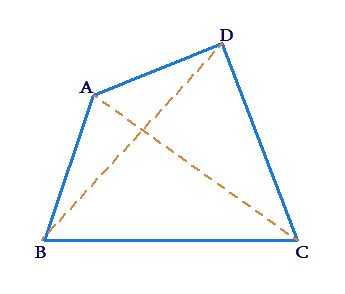How can we find its area?

Let us consider a number of different scenarios:

1. The length of the sides and one diagonal are known, Suppose that for the quadrilateral above, the lengths of the four sides and the diagonal AC  are known. In  this case, we can evaluate the areas of $$\Delta ABC$$ and $$\Delta ADC$$ separately and add them to obatain the area of the quadrilateral .
2. The length of one diagonal and the lengths of the perpendiculars from opposite vertices to this diagonal are known.

Consider the following figure: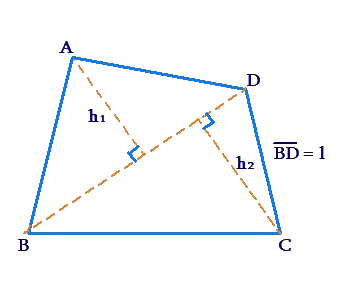We have :

\begin{align}&\rm{area}\left( {ABCD} \right) \;= area\left( {\Delta ABD} \right) + area\left( {\Delta CBD} \right)\\ &\qquad \qquad \qquad\;= \frac{1}{2} \times l \times {h_1} + \frac{1}{2} \times l \times {h_2}\\ &\qquad \qquad \qquad\;= \frac{1}{2} \times l \times \left( {{h_1} + {h_2}} \right)\end{align}

3. The diagonals are perpendicular and their lengths are known.

Consider the following figure: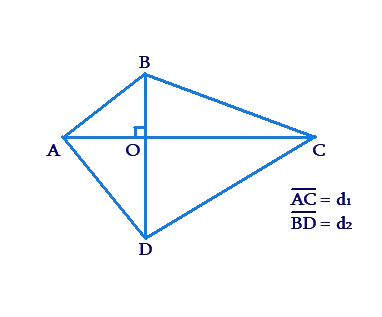We have:

\begin{align}&\rm{area}\left( {\Delta ABC} \right) = \frac{1}{2} \times AC \times BO\\&\rm{area}\left( {\Delta ADC} \right) = \frac{1}{2} \times AC \times DO\\&{\rm{Adding\;the\;two,\;we\;have}}\\&{\rm{area}}\left( {ABCD} \right)\quad= \frac{1}{2} \times AC \times \left( {BO + DO} \right)\\&\qquad\qquad\qquad\quad= \frac{1}{2} \times AC \times BD\\& \qquad\qquad\qquad\quad= \frac{1}{2} \times {d_1} \times {d_2}\end{align}

Thus, if the diagonals of a quadrilateral  are perpendicular, its area is given by half of the product of its diagonal lengths.

Example 1: Consider the following figure: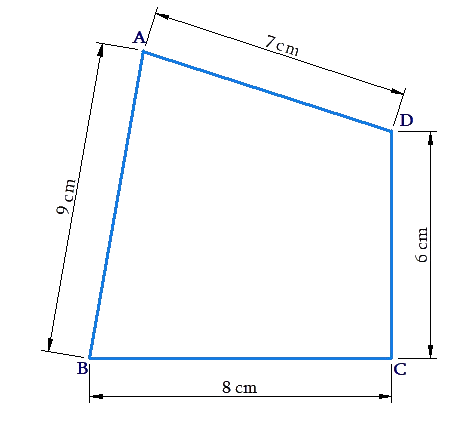Solution: Using the Pythagoras theorem, the length of BD will be $$\sqrt {{8^2} + {6^2}}$$ or 10 cm. Now, we find the areas of $$\Delta ABD$$ and $$\Delta CBD$$ separately .

• $$\rm{area}\,({\Delta ABD})$$

\begin{align}&S = \frac{{AB + BD + DA}}{2} = 13cm\\&\rm{Area}\,= \sqrt {13 \times \left( {13 - 9} \right) \times \left( {13 - 10} \right) \times \left( {13 - 7} \right)} \\&\qquad = \sqrt {13 \times 4 \times 3 \times 6} \\&\qquad \approx 30.6\, \rm{cm^2}\end{align}

• $$\rm{area}\,( {\Delta BCD})$$

\begin{align}&\rm{Area}\;= \frac{1}{2} \times BC \times CD\\&\qquad = \frac{1}{2} \times 8 \times 6\\&\qquad = 24\,\rm{cm^2} \end{align}

The total area of ABCD is thus approximately 54.6 cm2.

Example 2: The adjacent sides of a parallelogram have lengths 12 cm and 8 cm.If the distance between the shorter sides is 6 cm, what is the distance between the longer sides ?

Solution: Consider the following figure: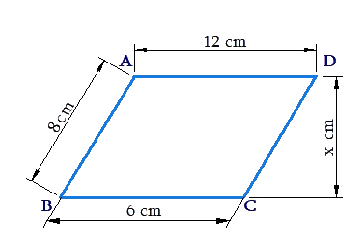We can explain the area of ABCD as either

•     $$\left( {BC \times x} \right)\; \rm{cm^2}$$

•     $$or{\rm{ }}\left( {AB \times 6} \right)\;\rm{cm^2}$$

Thus,

\begin{align}&BC \times x = AB \times 6\\&\Rightarrow 12x = 48\\&\Rightarrow X = 4\end{align}

The distance between the longer sides is 4 cm.

Example 3:  A  park has the following dimensions: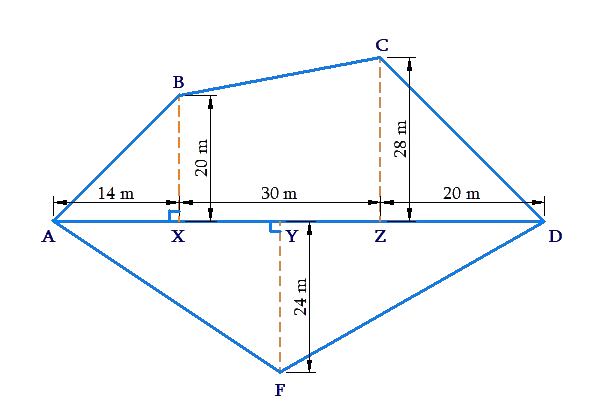Find its  total area.

Solution: We have:

\begin{align} & \text{area}\left( \Delta \,ABX \right)\text{=}\frac{\text{1}}{\text{2}}\times \text{14}\times \text{20=140}\,{{\text{m}}^{\text{2}}} \\ & \text{area}\left( \text{Trap}.\ BCZX \right)\text{=}\frac{\text{1}}{\text{2}}\times \left( \text{20+28} \right)\times \text{30} \\ & \qquad \qquad \qquad \qquad =720\,{{\text{m}}^{\text{2}}} \\ & \text{area}\left( \Delta \,CDZ \right)\text{=}\frac{\text{1}}{\text{2}}\times \text{20}\times \text{28=280}\,{{\text{m}}^{\text{2}}} \\ & \text{area}\left( \Delta \,ADF \right)\text{=}\frac{\text{1}}{\text{2}}\times AD\times FY \\ & \qquad \qquad \qquad \ =\frac{1}{2}\times 64\times 24=768\,{{\text{m}}^{\text{2}}} \\ \end{align}

By adding all these areas, we obtain the total area of the park as 1908 m2.

Example 4:  What  is the area of the following quadrilateral?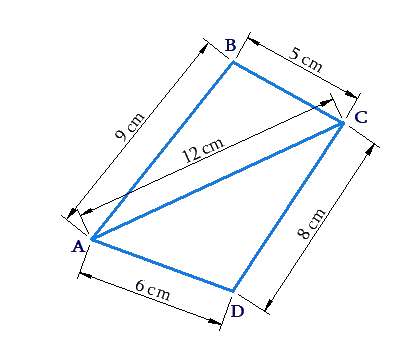Solution: We find the areas of both $$\Delta ABC$$ and  $$\Delta ADC$$ using the Heron’s formula.

• $$\Delta ABC$$

\begin{align}&\quad\qquad s = \frac{{AB + BC + CA}}{2} = 13 \;\rm{cm}\\&\quad \rm{Area} = \sqrt {13 \times \left( {13 - 9} \right) \times \left( {13 - 5} \right) \times \left( {13 - 12} \right)} \\&\qquad\quad= \sqrt {13 \times 4 \times 8 \times 1} \\&\qquad\quad{\rm{}} \approx 20.4\;\rm{cm^2}\end{align}

• $$\Delta ADC$$
\begin{align}&\qquad s = \frac{{AD + DC + CA}}{2} = 13\,\rm{cm}\\&\rm{Area} = \sqrt {13 \times \left( {13 - 6} \right) \times \left( {13 - 8} \right) \times \left( {13 - 12} \right)} \\&\qquad= \sqrt {13 \times 7 \times 5 \times 1} \\&\qquad \approx 21.3\; \rm{cm^2}\end{align}

The total area is thus approximately equal to 41.7cm2 .

Example 5: Consider  the following figure ABCD is a rhombus while DCEF is a trapezium.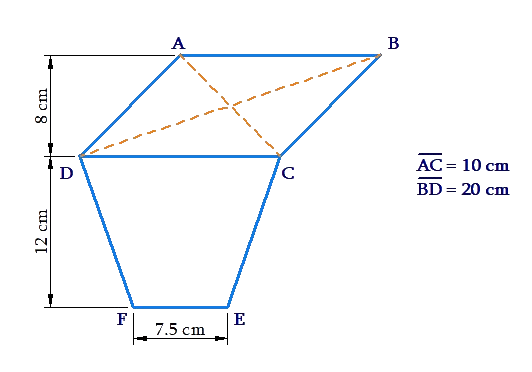What is the total area of the polygon ABCEFD?

Solution: We note that the area  of the rhombus ABCD is

$\frac{1}{2} \times AC \times BD = \frac{1}{2} \times 10 \times 20 = 100c{m^2}$

We can also write this same area as $$CD \times \left( {{\text{distance between AB and CD}}} \right)$$ .Thus,

\begin{align}&CD \times 8 = 100\\&\Rightarrow CD = 12.5\,\rm{cm}\end{align}

Now,

\begin{align} & \ \text{area}\left( trap\ DCEF \right)\text{=}\frac{\text{1}}{\text{2}}\times \left( CD\text{+}EF \right)\times \text{12} \\ & \qquad \qquad \qquad \quad \quad =\frac{1}{2}\times \left( 12.5+7.5 \right)\times 12 \\ & \qquad \qquad \qquad \quad \quad =120\,\text{c}{{\text{m}}^{2}}\end{align}

Thus, the total area is (100+120) or 220cm2.

Example 6:  Find the area of the following trapezium: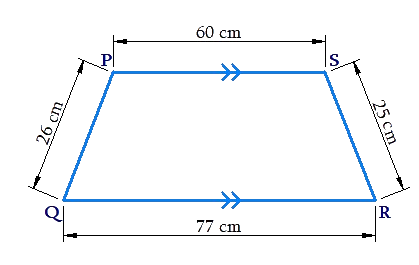Solution:Observe that  PS||QR. However, we do no know the distance between these parallels. How do we calculate the area in this case?

Through P, draw PX||SR meeting QR at X, and $$PY \bot QR$$, as shown: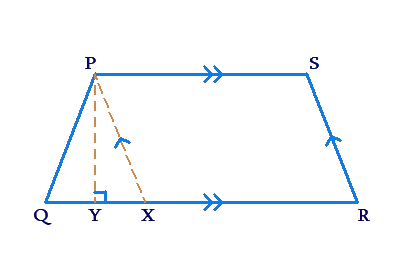Note that PSRX is a parallelogram, and thus, PX = SR=25 cm, .Also, XR = PS = 60 cm, and so,

$QX = QR - XR = 77 - 60 = 17 \,\rm{cm}$

Now, let us calculate the area of $$\Delta PQX$$ using Heron’s formula . We have:

$s = \frac{{PQ + QX + XP}}{2}\;=\;\frac{{26 + 17 + 25}}{2} = 34\, \rm{cm}$

Thus,

\begin{align}&\rm{area}\,\left( {\Delta PQX} \right) = \sqrt {34 \times \left( {34 - 26} \right) \times \left( {34 - 17} \right) \times \left( {34 - 25} \right)} \\&\qquad\qquad\qquad = \sqrt {34 \times 8 \times 17 \times 9} \\&\qquad\qquad\qquad = 204 \;\rm{cm^2}\end{align}

However, we can also express this area as $$\frac{1}{2} \times QX \times PY$$ .

Thus,

\begin{align}&\frac{1}{2} \times QX \times PY = 204\\&\Rightarrow \frac{1}{2} \times 17 \times PY = 204\\ &\Rightarrow PY = 24\; \rm{cm}\end{align}

Thus, the distance between the parallels of the trapezium is 24 cm,and hence, its area is

\begin{align}&\frac{1}{2} \times \left( {PS + QR} \right) \times PY\\& = \frac{1}{2} \times \left( {60 + 77} \right) \times 24\\ &= 1644\,\rm{cm^2}\end{align}

Mensuration
grade 9 | Questions Set 1
Mensuration
Mensuration
grade 9 | Questions Set 2
Mensuration